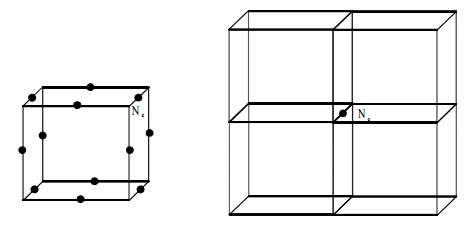Home | | Chemistry | Types of Cubic System

# Types of Cubic SystemTypes of Cubic System : i. Simple cubic ii. Body - centred cubic iii. Face - centred cubic Assignment of Atoms per unit cell in a cubic lattice

UNIT CELL

Unit cell is the smallest fundamental repeating portion of a crystal lattice from which the crystal is built by repetition in three dimension.

Characteristic parameters of a unit cell

Crystallographic axes : ox, oy, oz

Interfacial angles : a, b, g

Primitives (Intercepts) : a, b, cTypes of Cubic System

i.                        Simple cubic

ii.                        Body - centred cubic

iii.                        Face - centred cubicAssignment of Atoms per unit cell in a cubic lattice

Simple Cubic

In a simple cubic where atoms are present at the corners only, each atom at the corner is shared equally by eight other unit cells. Hence the contribution of each atom to the unit cell is 1/8.The total number of atoms per unit cell = Nc /8 = 8/8 = 1

Nc is the number of atoms at the corners.

Fcc

A face atom is shared equally between two unit cells and therefore a face atom contributes only (Nf/2) to the unit cell.

The number of atoms per unit cell in fcc = Nc/8 + Nf/2 = (8/8) + (6/2) = 4

Nf = Number of atoms at the faces.

Nf = Number of  atoms at the facesBCC

In a bcc lattice, the body centred atom belongs exclusively to the unit cell.

The total number of atoms per unit cell in bcc

= (Nc/8) + (Ne/4)  = (8/8) + (12/4) = 4

Nb = Number of atoms inside the body

An Edge atom (or) Edge centred

An edge atom and edge centred is common to four unit cells and there are twelve edges of the unit cell. The contribution from each edge atom is therefore 1/4. The number of atoms per unit cell in edge centre.

= (Nc/8) + (Ne/4)  = (8/8) + (12/4) = 4

Ne = Number of atoms at the edge centreStudy Material, Lecturing Notes, Assignment, Reference, Wiki description explanation, brief detail
11th 12th std standard Class Organic Inorganic Physical Chemistry Higher secondary school College Notes : Types of Cubic System |